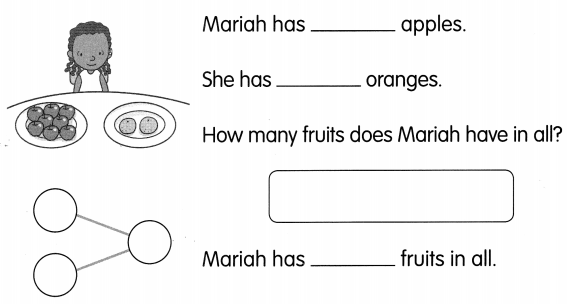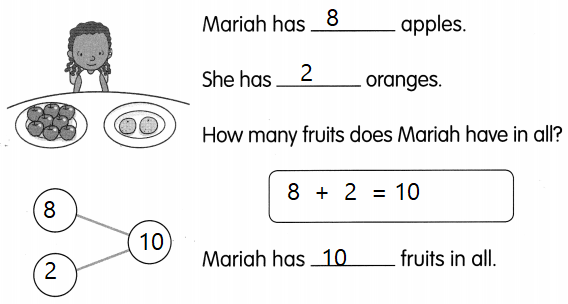This handy Math in Focus Grade 1 Workbook Answer Key Chapter 3 Practice 4 Real-World Problems: Addition detailed solutions for the textbook questions.

Solve.

Example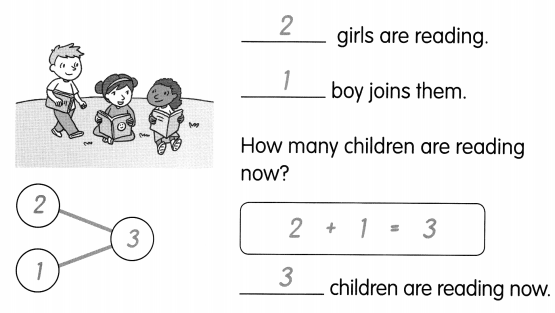Question 1.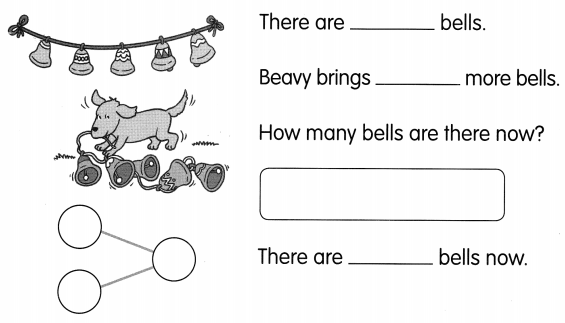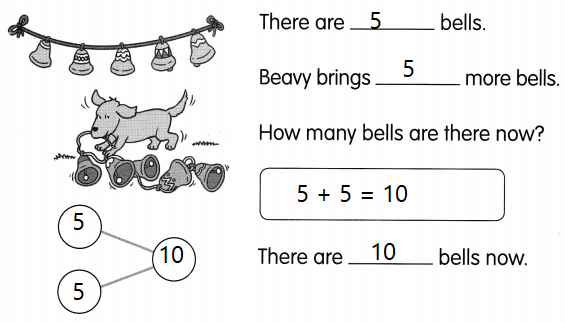Explanation:
Number of bells are 5
A bog bring 5 more bells
5 + 5 = 10
The sum of 5 and 5 is 10

Question 2.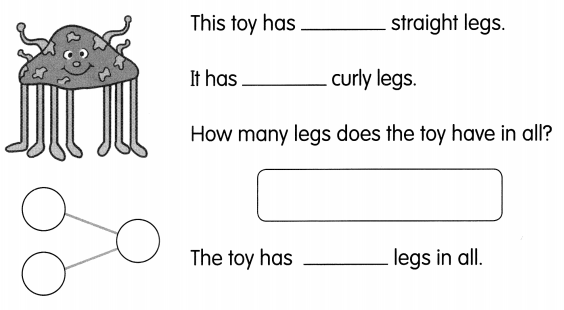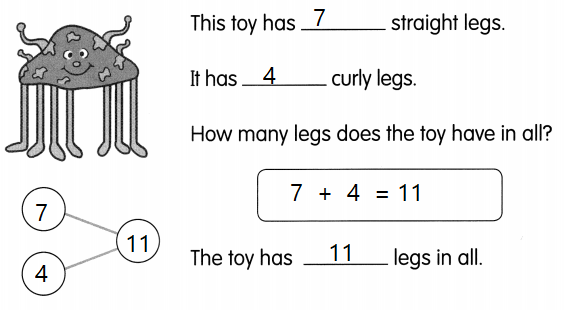Explanation:
An octopus has 8 legs
and 4 more legs that are curly
7 + 4 = 11
The sum of 7 and 4 is 11

Question 3.